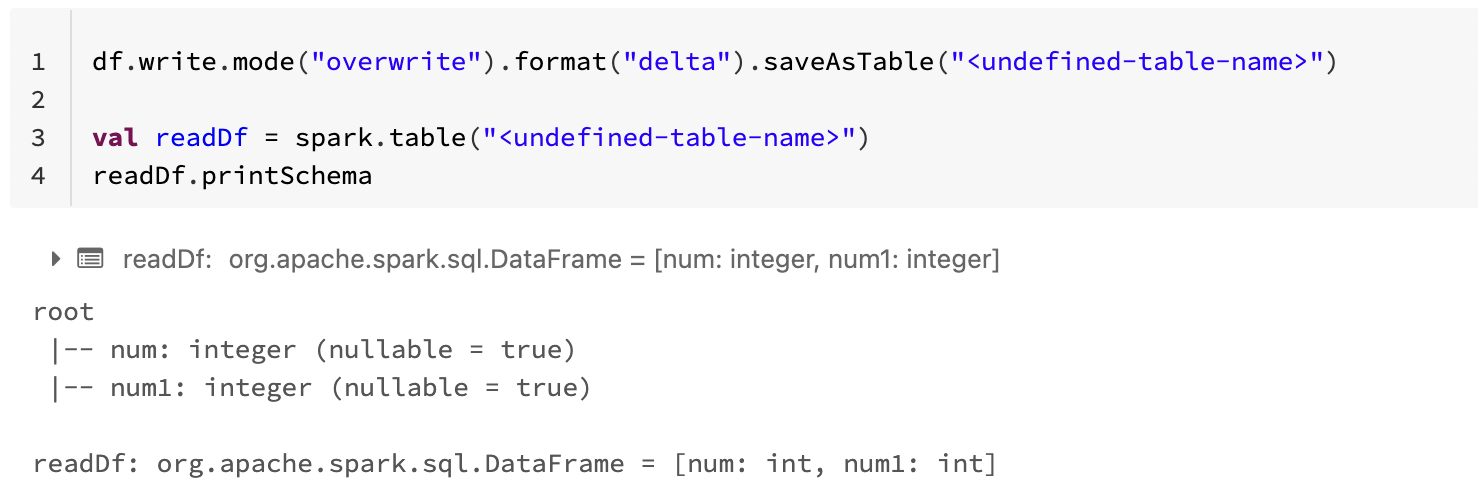# Set nullability when using SaveAsTable with Delta tables

Learn how to create a Delta table with the nullability of columns set to false.Written by

Last published at: October 14th, 2022

When creating a Delta table with saveAsTable, the nullability of columns defaults to true (columns can contain null values). This is expected behavior.

In some cases, you may want to create a Delta table with the nullability of columns set to false (columns cannot contain null values).

# Instructions

Use the CREATE TABLE command to create the table and define the columns that cannot contain null values by using NOT NULL.

For example, this sample code creates a Delta table with two integer columns. The column named null can contain null values, but the column named null1 cannot contain null values because it was created with NOT NULL.

```%sql

CREATE TABLE <table-name> (
num Int,
num1 Int NOT NULL
)
USING DELTA```

Now that we have the Delta table defined we can create a sample DataFrame and use saveAsTable to write to the Delta table.

This sample code generates sample data and configures the schema with the isNullable property set to true for the field num and false for field num1. This sample data is stored in a newly created DataFrame.

For the final step, saveAsTable is used to write the data to the table we previously created.

```import org.apache.spark.sql.types._
val data = Seq(
Row(1, 3),
Row(5, 7)
)

val schema = StructType(
List(
StructField("num", IntegerType, true),
StructField("num1", IntegerType, false)
)
)

val df = spark.createDataFrame(
spark.sparkContext.parallelize(data),
schema
)

df.write.mode("overwrite").format("delta").saveAsTable("<table-name>")```

If you read the table schema, num allows for null values while num1 does not allow null values.```root
|-- num: integer (nullable = true)
|-- num1: integer (nullable = false)

```

Delete

### Warning

If you do not configure the nullability of your columns by creating a table in advance and instead try to write data to an undefined table, the nullability of all columns defaults to true. The DataFrame scheme is ignored in this case.

For example, if you skip table creation and just try to write the data to a table with saveAsTable, and then read the schema, all columns are defined as being nullable.